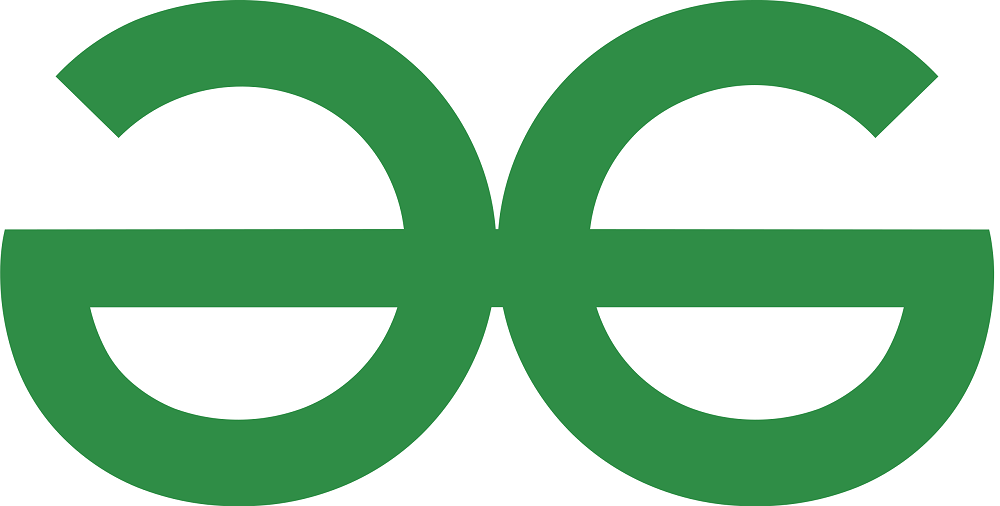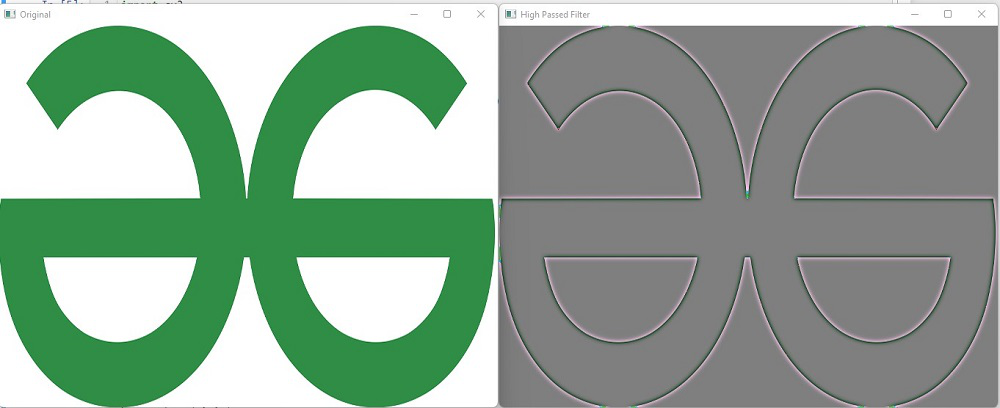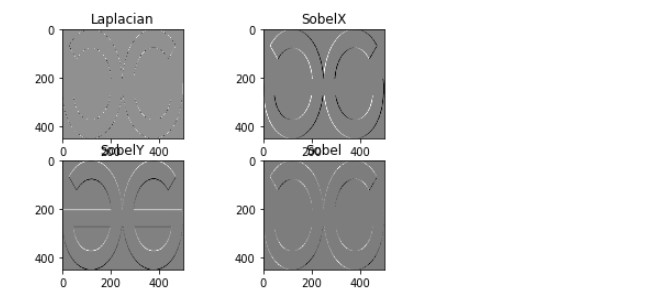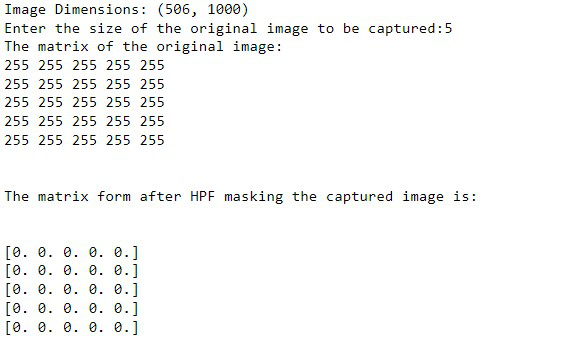# Implement Photoshop High Pass Filter (HPF) using OpenCV in Python

• Difficulty Level : Medium
• Last Updated : 03 Jun, 2022

In this article, we will discuss how to implement photoshop High Pass Filter (HPF) image in Python OpenCV.

## High Pass Filter

When we talk about Digital Signal Processing or Digital Image Processing Filters are the most fundamental concept. A Filter or a Digital Filter is used to sifting out the unwanted frequency response from the signals. A filter can be used to fulfill two main tasks in Digital Signal processing and those are signal separation and signal restoration. Signal separation is basically a noise separation and noise attenuation from the input signals. This process is mainly used in EKG, An electrocardiogram monitor to filter the breathing and heartbeat of the mother. Signal Restoration is a process when the input signal is distorted by any means. This is mainly used in deblurring an image. We shall look at what High Pass Filter is mainly used for.

A High Pass Filter(HPF) main advantage is used to sharpen the image by attenuating the low frequency. When the impulse response or signal is passed through a high pass filter, an HPF mainly allows high frequencies to pass through. As High pass filters are used for sharpening the images, the frequency obtained is less compared to the cut-off frequency(ωc). In OpenCV and in digital image processing we also use HPF functionality to find the edges in an image.

## Method 1: High Pass Filter(HPF) in Python OpenCV

Here we are going to perform HPF using OpenCV in Python

Used images:Now that we have an image, using the Python OpenCV module we shall read the image.

Given the size of the image, we can also resize the shape this step is completely optional. While resizing the image you can pass an interpolation so that the image maintains its quality. You are free to choose cv2.INTER_BITS, cv2.INTER_CUBIC or cv2.INTER_LINEAR methods for resizing interpolation.

img = cv2.resize(img,(width,height),interpolation=cv2.INTER_BITS)

The next step is to blur the image. The reason to blur the image is to add smoothening effect to an image. By blurring the image we can filter the unwanted noise from the image. In the OpenCV library, we widely use the Gaussian Filter. It employs the technique “kernel convolution”.

Note: 127 is added after subtracting the image with a blurred image to add the greyish look. We shall use Gaussian Blur to blur the image.

hpf = img – cv2.GaussianBlur(img,(21,21),3)+127

## Python3

 `import` `cv2`   `img ``=` `cv2.imread(``"naruto.jpg"``)` `img ``=` `cv2.resize(img, (``680``, ``520``), ` `                 ``interpolation``=``cv2.INTER_CUBIC)`   `# subtract the original image with the blurred image` `# after subtracting add 127 to the total result` `hpf ``=` `img ``-` `cv2.GaussianBlur(img, (``21``, ``21``), ``3``)``+``127`   `# display both original image and filtered image` `cv2.imshow(``"Original"``, img)` `cv2.imshow(``"High Passed Filter"``, hpf)`   `# cv2.waitkey is used to display` `# the image continuously` `# if you provide 1000 instead of 0 then ` `# image will close in 1sec` `# you pass in milli second` `cv2.waitKey(``0``)` `cv2.destroyAllWindows()`

Output:## Method 2: Using Sobel and Laplacian

Python OpenCV supports Sobel and Laplacian implementation.

Syntax for Laplacian:  laplacian=cv2.Laplacian(gray,cv2.CV_64F)

Syntax For SobelX: [dx=1 and dy=0]:  sobelx=cv2.Sobel(gray,cv2.CV_64F,1,0,ksize=7)

Syntax For SobelY: [dx=0 and dy=1]:  sobely=cv2.Sobel(gray,cv2.CV_64F,dx=0,dy=1,ksize=7)

Note: cv2.CV_64F denotes the depth of the image

## Python3

 `import` `cv2` `import` `numpy as np` `import` `matplotlib.pyplot as plt`   `# read image` `img ``=` `cv2.imread(``"naruto.jpeg"``)`   `# resize image` `img ``=` `cv2.resize(img, (``500``, ``450``), interpolation``=``cv2.INTER_CUBIC)`   `# convert image to gray scale image` `gray ``=` `cv2.cvtColor(img, cv2.COLOR_BGR2GRAY)`   `# apply laplacian blur` `laplacian ``=` `cv2.Laplacian(gray, cv2.CV_64F)`   `# sobel x filter where dx=1 and dy=0` `sobelx ``=` `cv2.Sobel(gray, cv2.CV_64F, ``1``, ``0``, ksize``=``7``)`   `# sobel y filter where dx=0 and dy=1` `sobely ``=` `cv2.Sobel(gray, cv2.CV_64F, ``0``, ``1``, ksize``=``7``)`   `# combine sobel x and y` `sobel ``=` `cv2.bitwise_and(sobelx, sobely)`   `# plot images` `plt.subplot(``2``, ``2``, ``1``)` `plt.imshow(laplacian, cmap``=``'gray'``)` `plt.title(``'Laplacian'``)`   `plt.subplot(``2``, ``2``, ``2``)` `plt.imshow(sobelx, cmap``=``'gray'``)` `plt.title(``'SobelX'``)`   `plt.subplot(``2``, ``2``, ``3``)` `plt.imshow(sobely, cmap``=``'gray'``)` `plt.title(``'SobelY'``)`   `plt.subplot(``2``, ``2``, ``4``)` `plt.imshow(sobel, cmap``=``'gray'``)` `plt.title(``'Sobel'``)`   `plt.show()`   `cv2.waitKey(``0``)` `cv2.destroyAllWindows()`

Output:## Method 3: Manually Perform High Pass Filter On Image

Since we have already defined the matrix method, let us manually filter our image data. By keeping a reference of the Laplacian Matrix let’s display the pixel matrix format of an image after HPF masking.

## Python3

 `import` `cv2` `import` `numpy as np`   `img ``=` `cv2.imread(``'naruto.jpeg'``)` `gray ``=` `cv2.cvtColor(img, cv2.COLOR_BGR2GRAY)`   `# dimension of gray image is in 2D` `dimensions ``=` `gray.shape  ``# (height,weight)`   `print``(``"Image Dimensions:"``, dimensions)` `n ``=` `int``(``input``(``"Enter the size of the original image to be captured:"``))` `print``(``"The matrix of the original image:"``)`     `for` `i ``in` `range``(``0``, n):` `    ``for` `j ``in` `range``(``0``, n):` `        ``print``(gray[i][j], end``=``" "``)` `    ``print``()` `"""` `Apply below filter on image matrix` `0 -1 0` `-1 4 -1` `0  -1 0` `"""` `filter` `=` `np.zeros(shape``=``(n, n))` `for` `i ``in` `range``(``0``, n):` `    ``for` `j ``in` `range``(``0``, n):` `        ``filter``[i][j] ``=` `0``*``gray[i][j]``-``1``*``gray[i][j``+``1``]\` `        ``+``0``*``gray[i][j``+``2``]``-``1``*``gray[i``+``1``][j]``+``4` `*` `\` `            ``gray[i``+``1``][j``+``1``]``-``1``*``gray[i``+``1``][j``+``2``]``+``0``*``gray[i``+``2``][j] ``-` `\` `            ``1``*``gray[i``+``2``][j``+``1``]``+``0``*``gray[i``+``2``][j``+``2``]`   `print``(``"\n"``)` `print``(``"The matrix form after HPF masking the captured image is:"``)` `print``(``"\n"``)` `for` `hpf ``in` `filter``:` `    ``print``(hpf)`   `cv2.imshow(``"Image"``, gray)` `cv2.waitKey(``0``)` `cv2.destroyAllWindows()`

Output:My Personal Notes arrow_drop_up
Recommended Articles
Page :